Unsupervised learning for anomaly detection in stock options pricing

Link to the Github (notebook + the data).

Note: This post is part of a broader work for predicting stock prices. The outcome (identified anomaly) is a feature (input) in a LSTM model (within a GAN architecture)- link to the post.

1. Motivation

Options valuation is a very difficult task. To begin with, it entails using a lot of data points (some are listed below) and some of them are quite subjective (such as the implied volatility — see below) and difficult to calculate precisely. As an example let us check the calculation for the call’s Theta — θ:

where (among others) N(d1) and N(d2) are cumulative distribution functions for a standard normal distribution, respectively:

Another example of how difficult options pricing is, is the Black-Scholes formula which is used for calculating the options prices themselves. The formula for calculating the price of a European call option with maturity in t and current price of S0 is:

Second, the Black-Scholes model, also known as binomial tree, requires a lot of assumptions to be met in order for the model to be accurate. These rules, however, more often than not, cannot be met in real life. Some of them include:

• the model assumes that the underlying volatility (σ) is constant over the life of the option and stays unaffected by changes in the underlying stock price levels. Many times the volatility changes with changes in the Strike price — the higher the difference between Strike and the underlying price, the higher the volatility. This phenomenon is called the Volatility smile (refer to the chart below),
• assumption of a constant risk-free rate of return (something difficult to anticipate as the global economy changes every day),
• the model does not account for liquidity risk and additional fees/charges,
• it assumes that stock returns follow lognormal distribution (meaning that the model ignores the possibility of large price deviations, surges or drops, something that can easily be observed in real trading),
• it assumes no dividend payout. Dividend payouts change the current valuation of the stock, which in turn should change the options price,
• the model is applicable only for European options.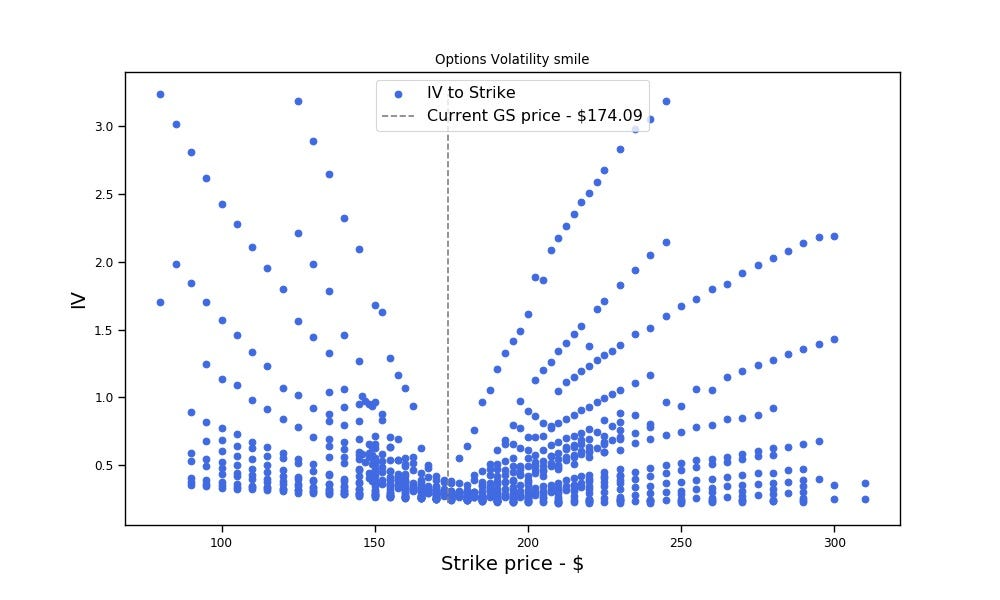Not a perfect smile at this example, but we only used data for one day.

The fact that the aforementioned assumptions can rarely be met in real-life is exactly why anomalies can be observed. This, in turn, creates a lot of opportunities we can explore and exploit with machine/deep learning, such as arbitrage trading.

Note : Knowledge of the options greeks is important in order for us to fully understand whether the anomaly detections works (and how). So we will briefly cover them:

• Implied volatility -σ: The implied volatility is a measure estimate of how much the price could change. A higher figure means that traders believe the option could make a large change. Basically, just a volatility index.
• Delta: δ measures how much the option price would change in relation to changes in the underlying stock price. A delta of 0.5 means the option would change 50 cents for every 1 dollar the stock moves (δ is the first derivative of the price).
• Gamma: γ measures how fast the δ will change when the stock price changes. A high number means this is a very ‘active’ option, and could gain or loss value quickly (this is the second derivative of the price).
• Theta: θ measures how fast the option is losing value per day due to time decay. As the expiration day arrives, the theta increases.
• Vega: The vega measures how sensitive the option price is to change in the implied volatility. Options that are out of the money, or have a long time until expiration are more sensitive to a change in implied volatility,
• Rho: rho is the rate at which the price of a derivative changes relative to a change in the risk-free rate of interest. (we don’t have data for Rho, tho).

So, back to anomalies detection.

2. Data

Options data can be found at historicaloptiondata.com. It would cost you \$1–2K but the data is rich as well ordered.

`# Filtered options data for Goldman Sachsoptions_df = pd.read_csv('option_GS_df.csv', parse_dates=["Expiration", ' DataDate'])`
`# Let's create Maturity and Spread featuresoptions_df['date_diff'] = (options_df['Expiration'] - options_df[' DataDate']).dt.daysoptions_df['Spread'] = options_df['Ask'] - options_df['Bid']`

The data we will use for this post is the daily quotes for Goldman Sachs options for Jan 5, 2016. It contains the pricing (plus volatility and greeks) for different maturities and exercise prices created on that date. The current (GS) price at that day — \$174.09. There are 22 features and 858 rows (each row is the pricing and greeks for a different Exercise and Maturity as of Jan 5, 2016). Check the Github repo for more info — link at the top.

`options_df.shape`
`output >>> (858, 22)`

What features do we have?

`print(', '.join(x for x in options_df.columns))`
`output >> UnderlyingPrice, OptionSymbol, Type, Expiration,  DataDate, Strike, Last, Bid, Ask, Volume, OpenInterest, T1OpenInterest, IVMean, IVBid, IVAsk, Delta, Gamma, Theta, Vega, AKA, date_diff, Spread`

Basically all features we need are available. Let’s visualise the data in a pair-plot, where:

• the features we plot against each other are the Strike, Bid, Delta, Gamma, Vega, and the Spread (we won’t plot every feature),
• the hue is the option’s type (call or put),
• the diagonal, of course, is the distribution of each feature.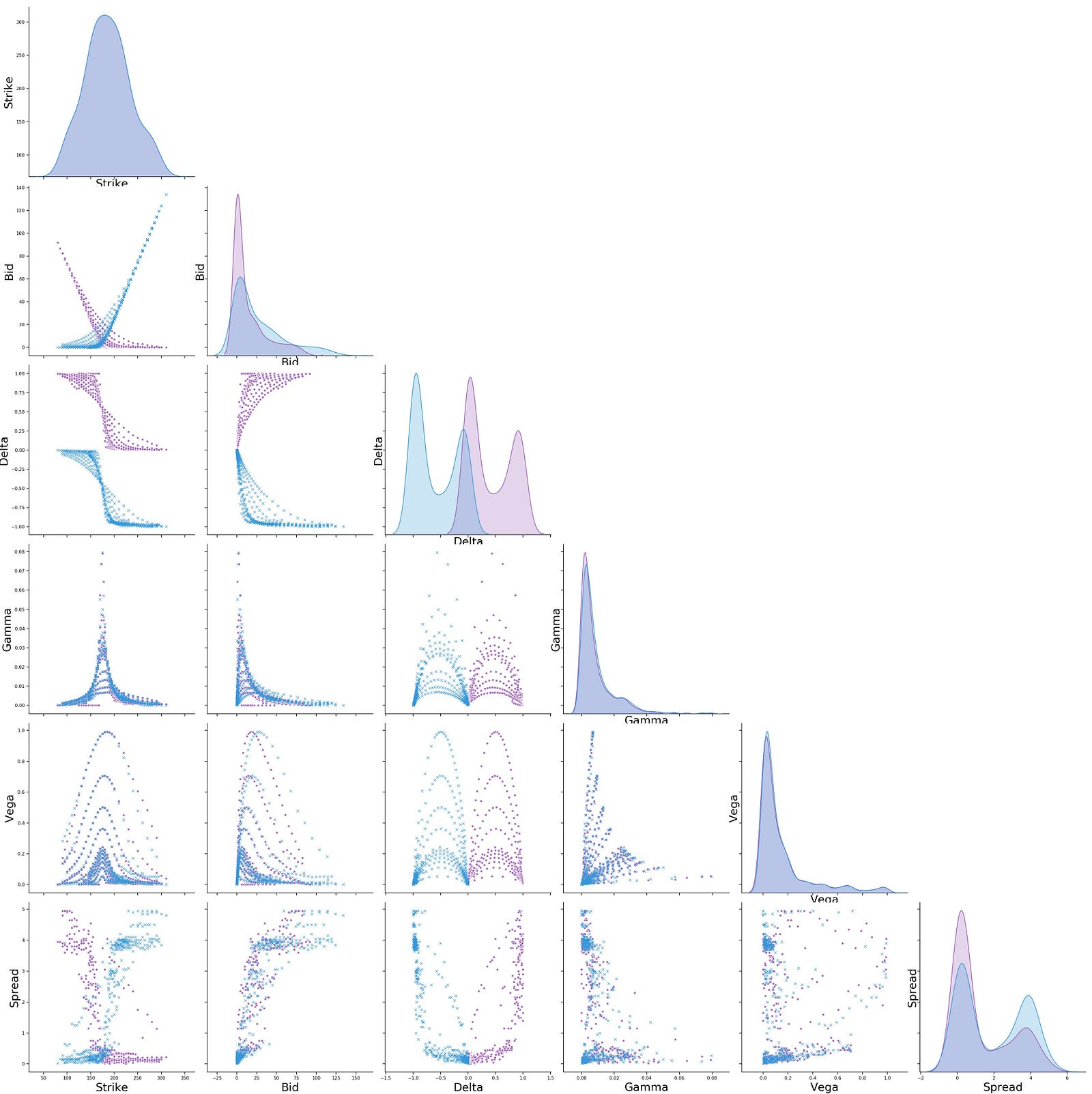Goldman Sachs options data for 2016/01/05. The purple-ish color represents the calls, and the blue — the puts.

3. Unsupervised learning for finding outliers (anomaly)

What would be an anomaly? Anomaly in our case (and in general) would be any mismatch in the logic of the options. For example, the Bid (or Ask) prices of two call options that have the same Strike price but, say, 1–2 days difference in the Exercise day should be almost identical (unless there is something unusual, which should, probably, be somehow accounted for in the greeks). So, a big difference in the Bid prices of these two options would not be ‘normal’. Or, for instance, high Theta or small Vega on an (OTM) option with long time to expiration. And so on.

Note: Again we will skip the technical and mathematical aspects behind options pricing (such as stochastic processes, Brownian motion, and diffusion equations). Rather, we try to see whether we can use machine learning to approximate all these math formulas using data (data-driven approach as opposed to a model-driven approach). Probably the results will not be as accurate as the original formulas, but will be less computationally intensive. Or maybe, on the other hand, machine learning can ‘learn’ how to perform financial modelling (such as options pricing) even better than the financial math, as we can incorporate a lot of new approaches and data in the models, allowing the models to uncover patterns and correlations hidden to humans.

For the purpose of anomaly detection we will use Isolation Forest.

Let’s visualise the options data for one trading day (2016/01/05) and try to see if we can visually identify anomalies. Several things look suspicious -these might (or not) be anomalies:

• in Chart 2 — it is strange to have a put contract with much smaller delta than the contracts next to it considering the contract has the same characteristics — Average of bid/ask, difference between current and strike price, very very close expiration date — it is the option at the bottom with blue color surrounded by gray color puts (it’s a little bit hidden). On the other side, there is a call with the same anomaly — the purple circle surrounded by gray ones.
• in Chart 3 — (on the right side) there are several call options (we know they are calls because call options have a positive delta — between 0 and 1) with significantly lower ranges of Theta — the circles have theta less than (-4.5), considering that the other options with same characteristics (close by) have thetas higher than (-1.5).

Our main features of interest will be the Bid/Ask price (or the feature we have created — the average of those) and the Spread (=Ask−Bid). Options of the same type, close Strike prices and expiration dates shouldn’t, in theory, have significantly different prices and spread.

The following four charts are made in Tableau.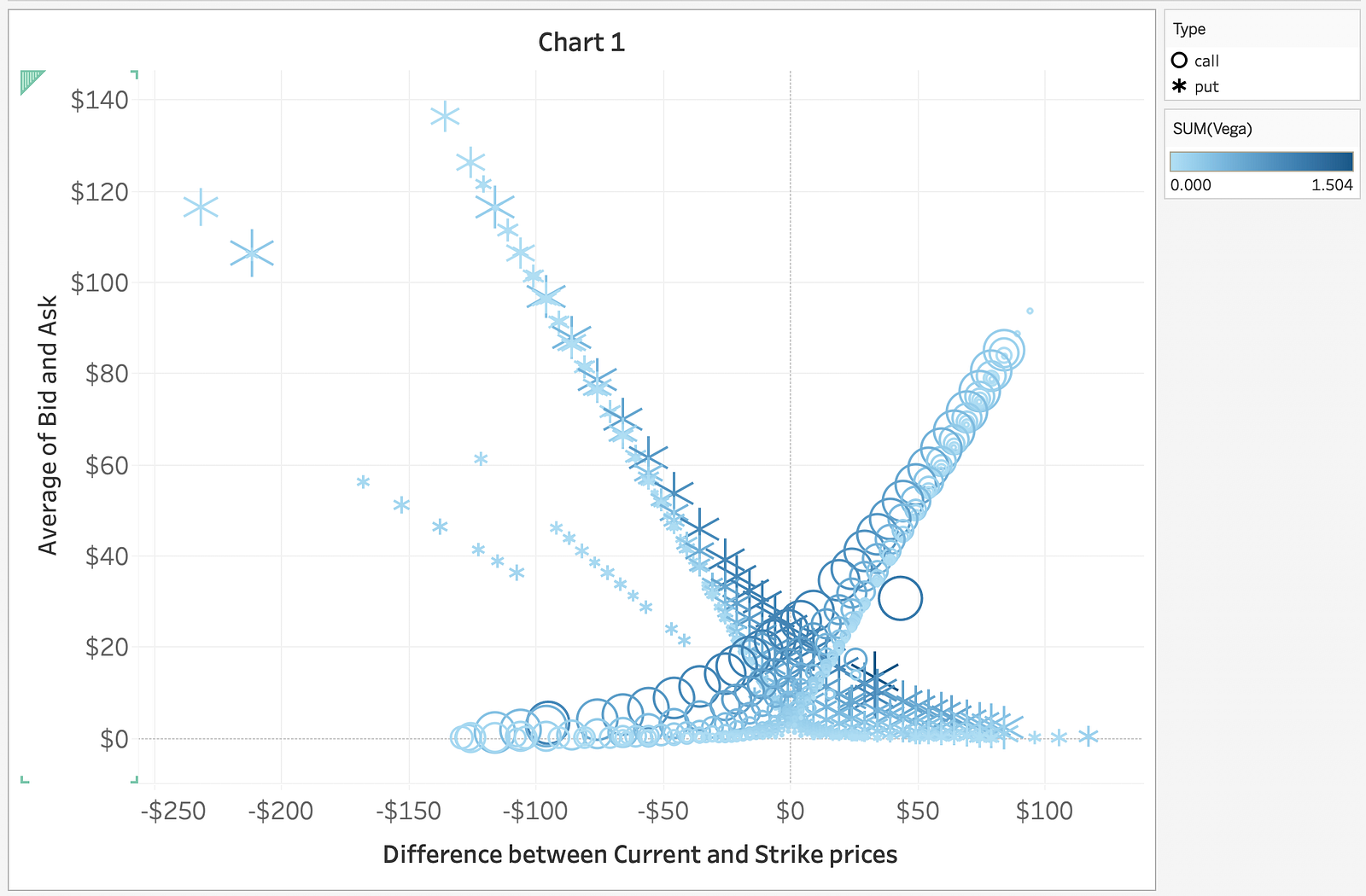GS options data (x-axis is the difference between the Current price and the Strike price, and the y-axis is the average of the Bid and Ask — basically the option’s price), where the circles represent calls and the stars are puts. The clustering color is based on Vega.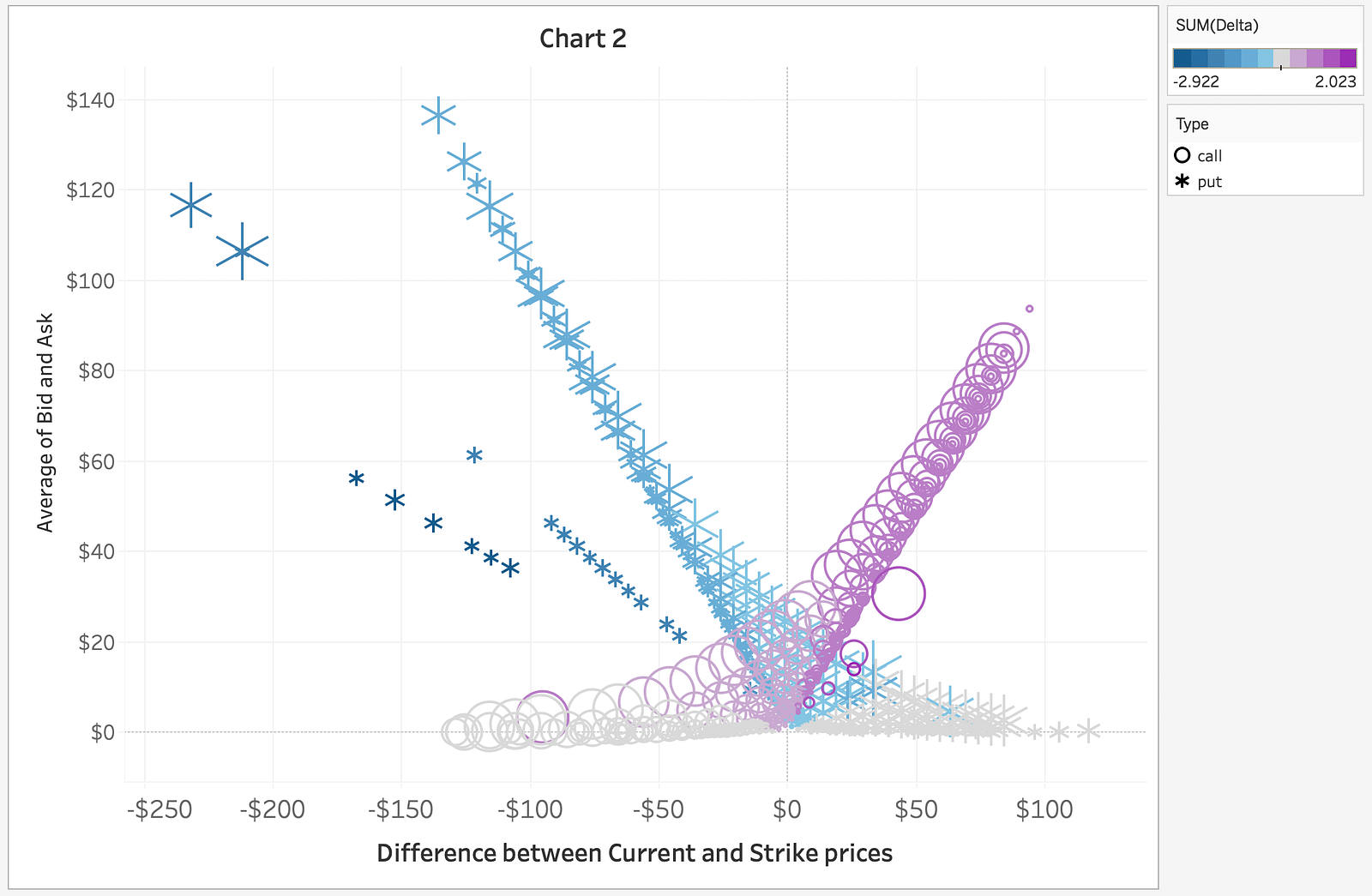GS options data (following the logic in Chart 1) but the clustering color is based on the Delta.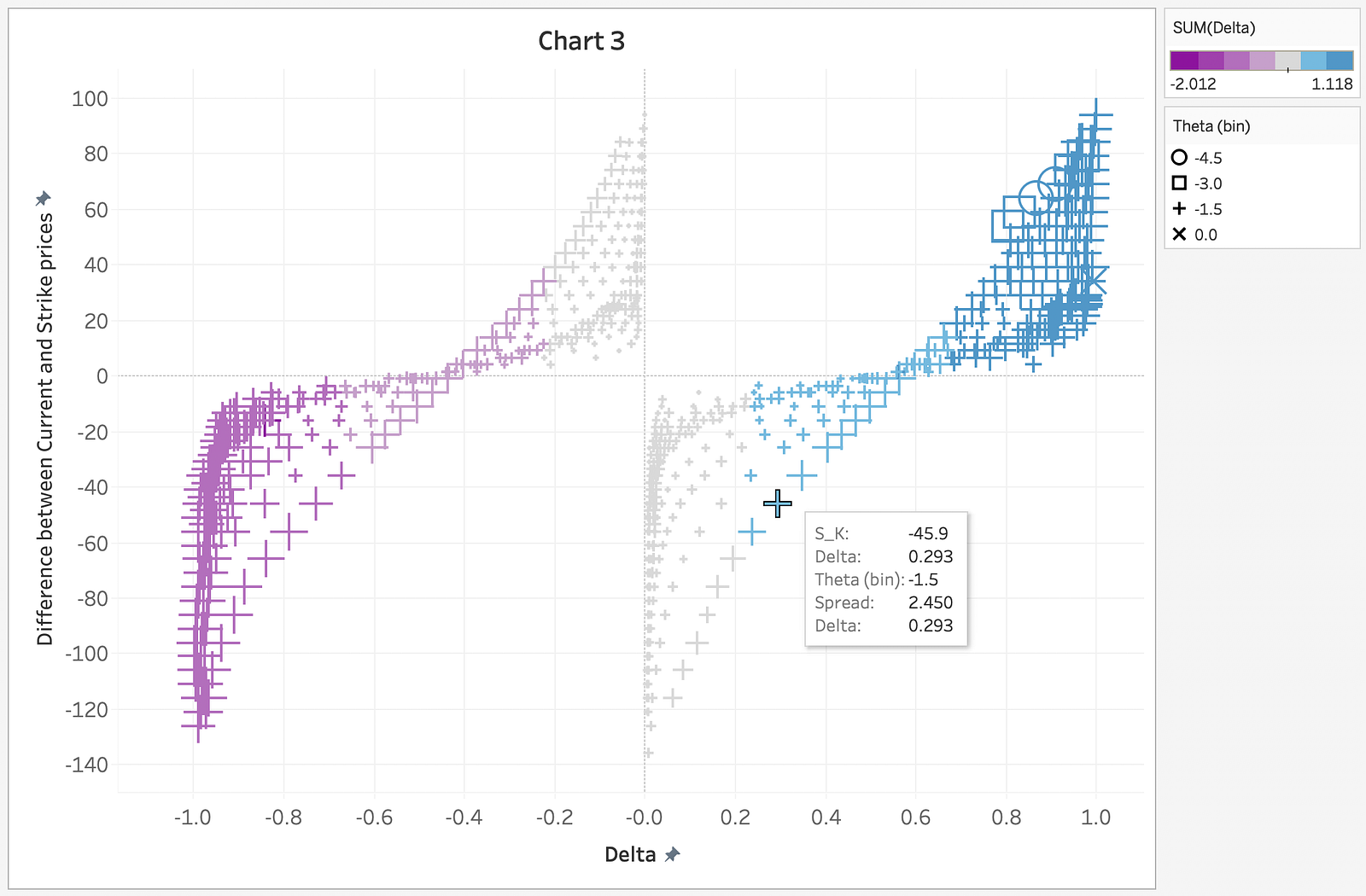GS options data (x-axis is the Delta, y-axis is the difference between Current price and Strike price). The clustering color is based on the Delta and the clustering shape (circle, square, plus, and x) is based on the Theta.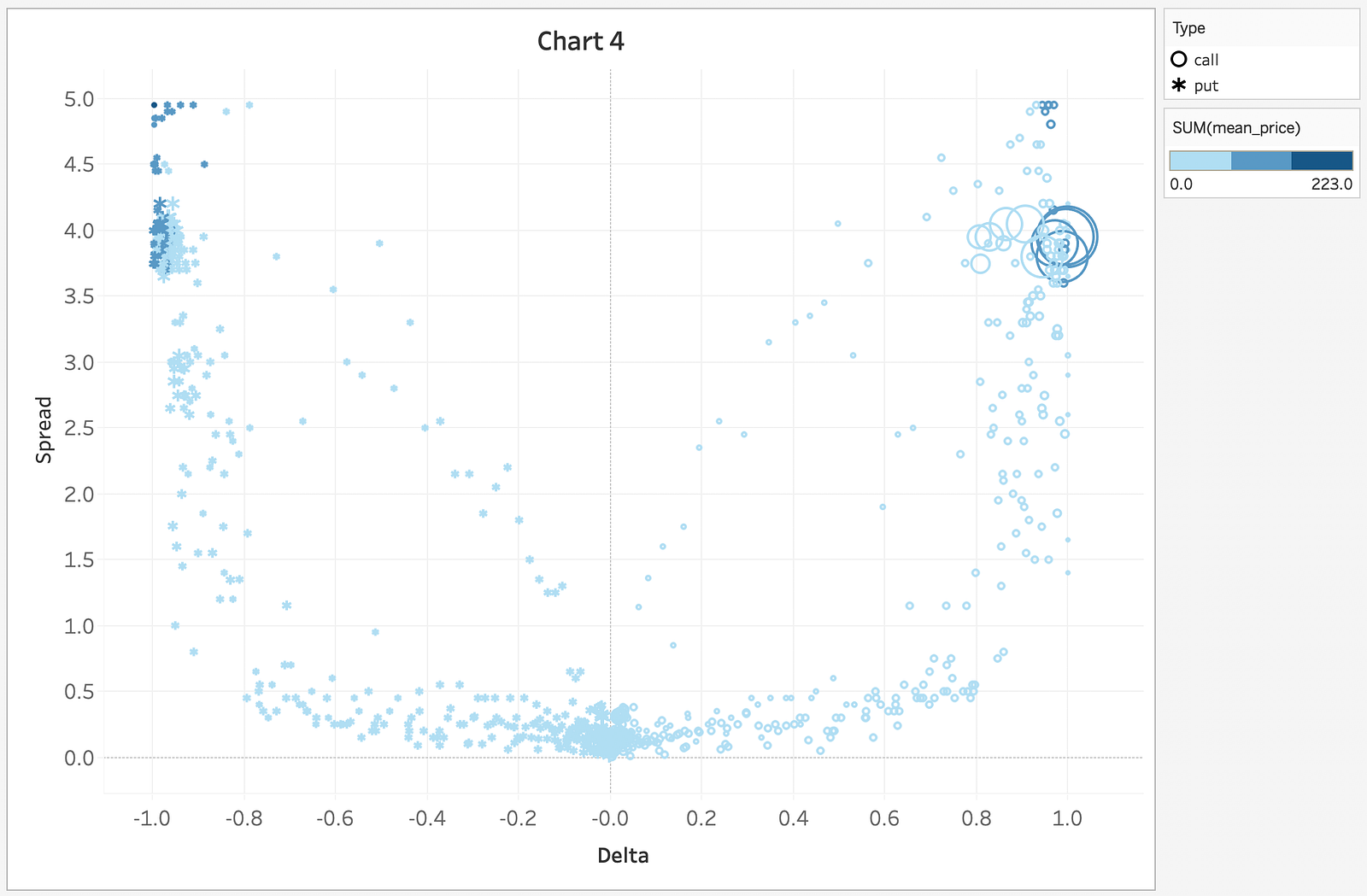GS options data (x-axis is Delta, y-axis is the Spread) where clustering color range comes from the mean price of Bid and Ask.

Ok, let’s jump into the anomaly detection. Our bet is that, through the data distribution, the algorithm will learn those optoins rules and manage to spot data points that don’t follow the ‘average’ distributions.

`it_X_train = options_df[['Strike', 'Delta', 'Gamma', 'date_diff']]it_X_train['s_k'] = options_df['UnderlyingPrice'] - options_df['Strike']it_X_train['b_a_mean'] = (options_df['Bid'] + options_df['Ask']) / 2it_X_train['b_a_mean'] = it_X_train['b_a_mean'].apply(lambda x: int(round(x, 0)))it_X_train['s_k'] = it_X_train['s_k'].apply(lambda x: int(round(x, 0)))`

We won’t use every feature for anomaly detection. The features we use are:

`print(', '.join(col_names[x] for x in it_X_train.columns))`
`output >>> Strike, Delta, Gamma, Difference between Date of valuation and Exercise date, Difference between Current and Strike prices, Average of Bid and Ask.`

Why do we use these features? We want to use features which (together) should follow the options logic described above. Broken logic among these six chosen features should constitute as a ‘strange’ contract.

The logic of the Isolation Forest is extremely simple:

`clf = IsolationForest(max_samples='auto', contamination=.025,\                      n_estimators=10, \                      random_state=19117,  max_features=it_X_train.shape)`
`clf.fit(it_X_train)y_pred_train = clf.predict(it_X_train)`

4. Results

Let’s visualise the outcome of the model. The x-axis is the difference between Current and Strike price and y-axis is the average of Bid and Ask (as Charts 1 and 2 above). The blue x’s and purple circles are put and call options, respectively, without anomaly in the features distributions. The others are anomalies.

These are the anomalies identified by the isolation forest. It was funny to find out (despite not being visible from the last chart as I couldn’t seem to fix the y-axis scales between python and Tableau) that the identified anomalies (in red and orange colors) are those we observed in the 4 charts above (I cross-referenced the indexes of each contract).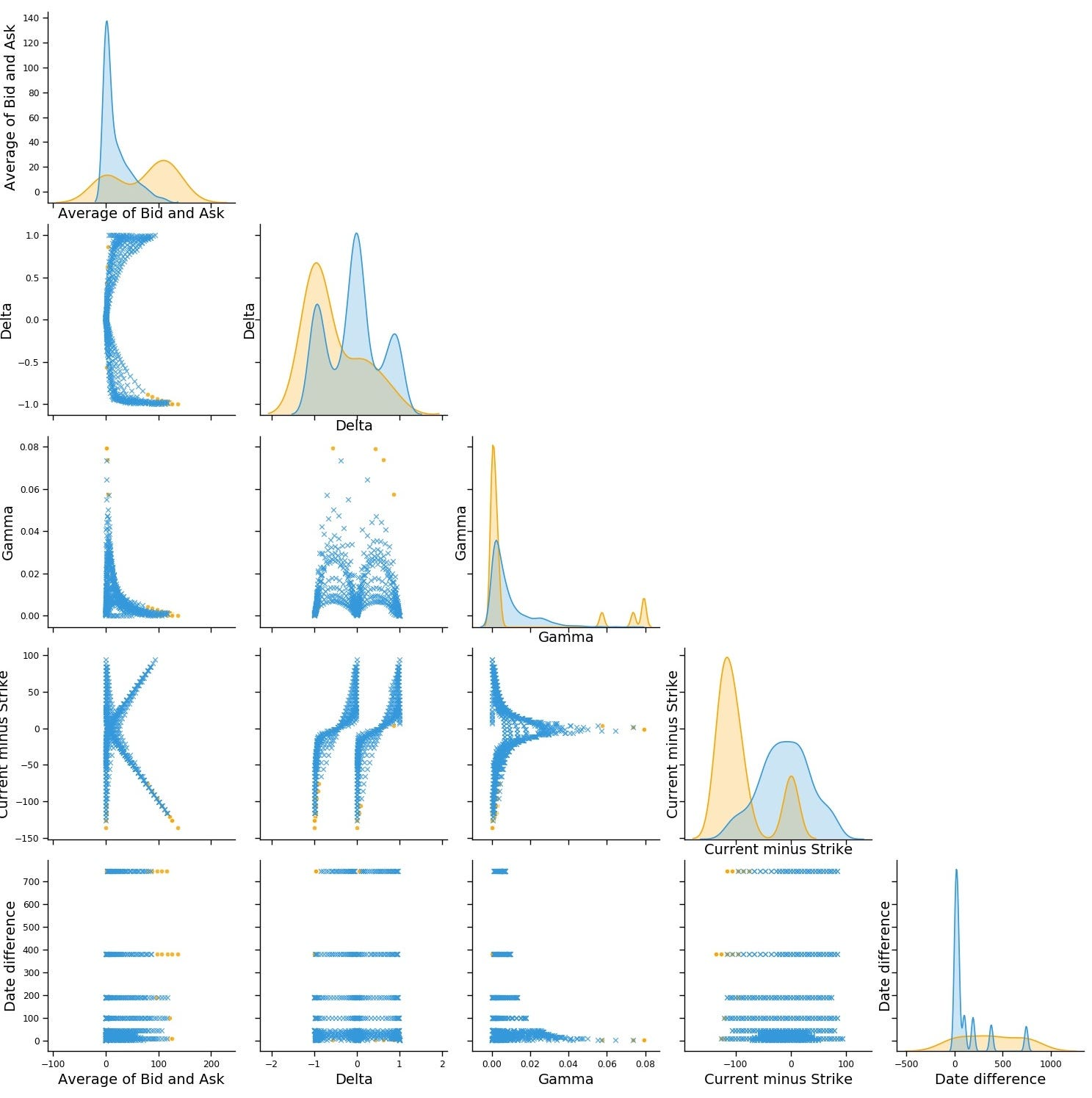Pair-plot between the features we used for anomaly detection. (Date difference is the number of days until expiration)

Several observations can be made about anomalous option pricing samples from this pair-plot:

• they have average price (average of Bid and Ask) higher than the other options,
• they are equally distributed across different maturities (despite the original data being distributed mainly around closer maturities),
• they Current minus Strike price is not normally distributed as the original data.

As mentioned above, we create anomaly detection on stock options pricing in order to use it as a feature for predicting Goldman Sachs’ stock price movements. How, and, at all, is anomaly in options pricing important for predicting stock price movements? Check it here. (still working on it, tho)